Courses
Courses for Kids
Free study material
Free LIVE classes
More

# Tangent-Secant TheoremLIVE
Join Vedantu’s FREE Mastercalss

## An Introduction to Tangent-Secant Theorem Formula

The tangent-Secant Theorem formula is a fundamental tool of Geometry found in Euclid’s Elements book. There are many methods to prove the theorem. Most important is using similar triangles. So, in this article, we will be discussing the proof of the Theorem in detail and its applications.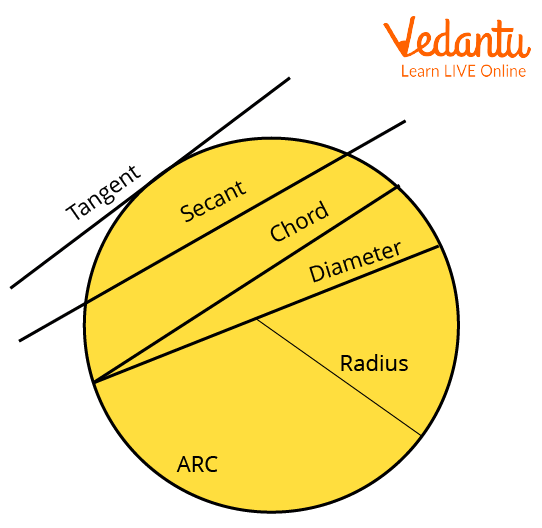Terms Associated with Circle

## History of Euclid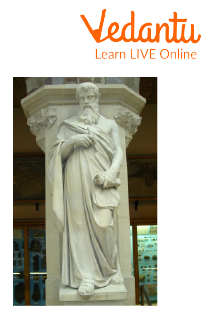Euclid

Name: Euclid

Born: Mid-4th century BC

Died: Mid-3rd century BC

Field: Mathematics

Nationality: Greek

## Statement of Tangent-Secant Theorem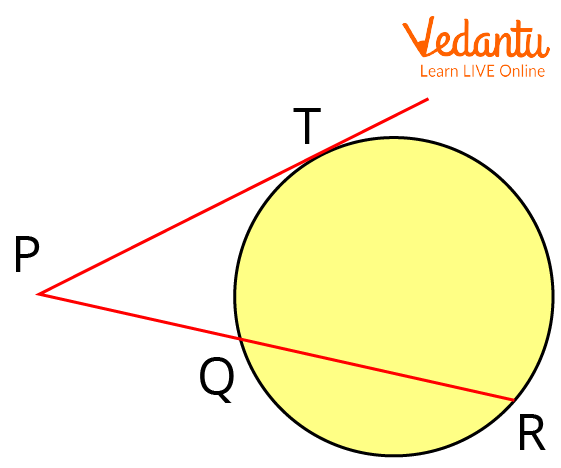Circle with PT tangent and PQR secant

For a circle with centre O, consider PT to be the tangent to the circle at the point from external point P and PQR to be the secant to the circle with points Q and R on the circle, then Tangent-Secant Theorem is defined as:

$P Q \times P R=P T^{2}$

## Proof of Secant Tangent Theorem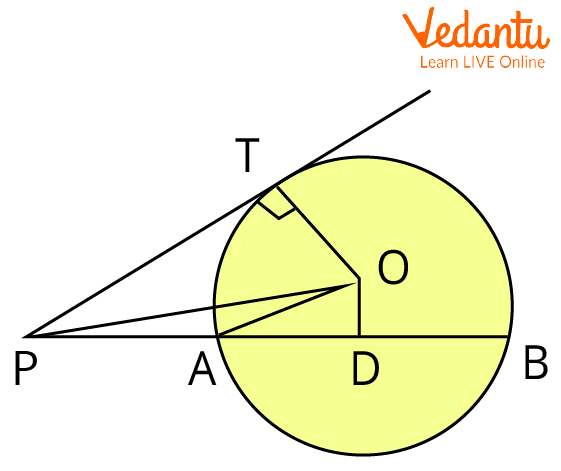Proof of the Tangent Secant Theorem

Given: PAB is secant to the circle with centre 0 and radius r. PT is tangent to the circle.

To prove: $P A \times P B=P T^{2}$

Construction: Draw $O D \perp A B$. Join $O P, O T$, and $O A$.

Proof:

Since $O D \perp A B$

$\therefore A D=D B \ldots(1)$ (Perpendicular from the centre to the chord bisects the chord)

$P A \times P B=(P D-A D)(P D \div B D)$

$\Rightarrow P A \times P B=(P D-A D)(P D+A D) \quad$ (Using 1)

$\Rightarrow P A \times P B=P D^{2}-A D^{2}$

In right $\triangle O P D$,

$O P^{2}=O D^{2}+P D^{2}$

$\Rightarrow P D^{2}=O P^{2}-O D^{2}$

$\therefore P A \times P B=\left(O P^{2}-O D^{2}\right)-A D^{2}$

$\Rightarrow P A \times P B=O P^{2}-\left(O D^{2}+A D^{2}\right)$

In right $\triangle O A D$,

$O A^{2}=O D^{2}+A D^{2}$

$\therefore P A \times P B=O P^{2}-O A^{2}$

$\Rightarrow P A \times P B=O P^{2}-O T^{2} \quad(\because O A=O T)$

In $\triangle O P T$,

$O P^{2}=P T^{2}+O T^{2}$

$\Rightarrow O P^{2}-O T^{2}=P T^{2}$

$\therefore P A \times P B=P T^{2}$

Hence proved.

## Limitations of Tangent-Secant Theorem

1. The tangent-Secant theorem doesn’t give any idea if the secant and tangent are not drawn from common points.

2. Tangent-Secant Theorem is only applicable in the case of 2-dimensional circles and not in 3-dimensional figure spheres.

## Applications of Tangent-Secant Theorem

• Tangent-Secant Theorem is of great significance. It is used in our day-to-day life such as school buildings, bridges, monuments etc.

• Monuments such as the Statue of Liberty and Pyramids are also based on concepts of secants and tangents.

### Solved Examples

1. In the given figure, $P Q=12 \mathrm{~cm}, P T=24 \mathrm{~cm}$, then find $R Q$.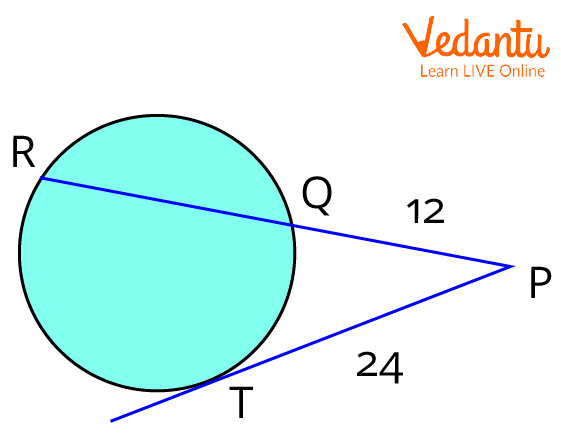PQ and Tangent PT is given

Ans:

Using Tangent Secant Theorem, we have

$P Q \times P R=P T^{2}$

Putting values, we get

$(12+x) \times 12=24^{2} \\$

$\Rightarrow(12+x) \times 12=576 \\$

$\Rightarrow(12+x)=48 \\$

$\Rightarrow x=48-12 \\$

$\Rightarrow x=36$

So, we get

$R Q=36 \mathrm{~cm}$

2. In a circle, the tangent is drawn from outside point $P$ to the circle at point $T$ and from the same point secant is drawn to the circle intersecting the circle at $Q$ and $R$, respectively, such that $P Q=25 \mathrm{~cm}, R Q=9 \mathrm{~cm}$, then find $P T$.

Ans:

According to the question, we get the following figure: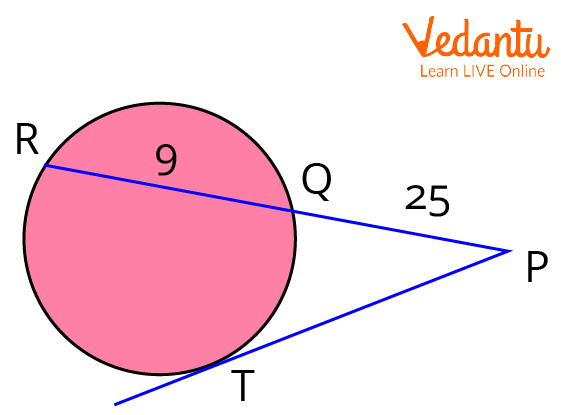Secant PQR is given

Using Tangent Secant Theorem, we have

$P Q \times P R=P T^{2}$

Putting values, we get

$(25+9) \times 25=P T^{2} \\$

$\Rightarrow P T^{2}=34 \times 25$

$\Rightarrow P T^{2}=850 \\$

$\Rightarrow P T=\sqrt{850} \\$

$\Rightarrow P T=29.154$

So, we get

$P T=12.154 \mathrm{~cm}$

3. In the given figure, $P R=84 \mathrm{~cm}, P T=42 \mathrm{~cm}$, then find $R Q$.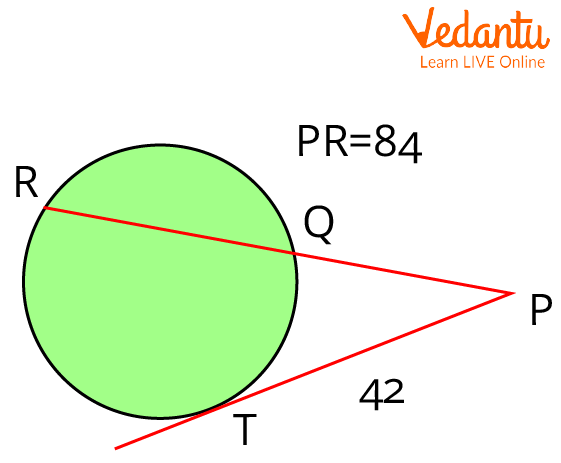Secant and tangent from a point are given

Ans:

Using Tangent Secant Theorem, we have

$P Q \times P R=P T^{2}$

Putting values, we get

$P Q \times 84=42^{2} \\$

$\Rightarrow P Q \times 84=1764 \\$

$\Rightarrow P Q=21$

Now,

$R Q=P R-P Q$

And,

$P Q=21 \\$

$\Rightarrow R Q=84-21 \\$

$\Rightarrow R Q=63 \mathrm{~cm}$

## Important Formulas to remember

• $P Q \times P R=P T^{2}$, where $\mathrm{PT}$ is tangent and $\mathrm{PQR}$ is secant to the circle with centre $O$ and radius $r$.

## Important Points to Remember

• A Tangent line touches the circle at only one point.

• A Secant line touches the circle at exactly two points.

## Conclusion

In the article, we have discussed the proof of the Tangent-Secant Theorem and its applications in detail. The Tangent Secant Theorem helps us in solving mathematical problems. In the world of art, architecture, and the growing demands of infrastructure, the tangent secant theorem places itself at the centre of its applications. In all, we can say that the theorem is of great importance.

Last updated date: 18th Sep 2023
Total views: 101.1k
Views today: 1.01k

## FAQs on Tangent-Secant Theorem

1. What do you mean by tangent at a point on a circle?

A tangent line to any plane curve is defined as the line that just touches the plane curve at a point. Here, the plane curve is a circle. So, the tangent at any point on a circle is the line which touches the circle at most and at only one point. Tangent is also sometimes referred to as slope in Geometry. We find the tangent at a point by finding the first derivative of the plane curve at the point at which we have to find the tangent on the curve.

2. What do you mean by the Secant line?

The word Secant is derived from the Latin word ‘Secure’ which means ‘to cut. Secant to a plane curve is the line segment which cuts the curve at a minimum of two points. In the case of a circle, the Secant Line is defined as the line which cuts the circle at exactly two points. Or we can also say that. The Secant Line to the circle is a chord of a circle which is extended outside the circle to become the secant of the circle.

3. What is the relationship between Secant and Tangent?

Secant and Tangents are different terms and have different meanings. We know that secant intersects the circle at exactly two points while the tangent interests the circle at most and at only one point while there is also a similarity between both terms. The value of the angle formed by two tangents or two secants or a secant and a tangent which intersect at a point outside the circle is equal to one-half the positive difference between the angle formed by intercepted arcs.